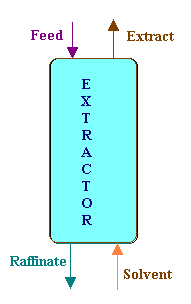### Extraction

A liquid containing 47.5% acetic acid and 52.5% water is to be separated by solvent extraction using isopropanol. The solvent used is 1.3 kg per kg of feed. The final extract is found to contain 82% acid on solvent free basis. The residue has 14% acid on solvent free basis. Find the percentage extraction of acid from the feed.

Calculations:

Basis: 1 kg of solvent free acid.Acetic acid in the feed = 0.475 kg

Water in the feed = 0.525 kg

Entering acid has to come out in the extract and residue (raffinate) phases.

Let as denote the streams with the following representation.

Feed: F

Extract: E

Residue: R

Solvent: S

And the mass fractions of acetic acid (on solvent free basis) in various streams:

Acetic acid in the feed: xF

Acetic acid in extract: xE

Acetic acid in residue xR

And the mass fraction of acetic acid in the solvent is zero, since the solvent is a pure one.

Balance on acetic acid:

FxF = ExE + RxR

1 x 0.475 = E x 0.82 + R x 0.14

0.475 = 0.82 E + 0.14 R   → 1

similarly writing the balance for water,

0.525 = (1 - 0.82) E + (1 - 0.14) R

0.525 = 0.18 E + 0.86 R   → 2

solving equations 1 and 2,

E = 0.493 kg, and

R = 0.507 kg.

Amount of acetic acid in the extract = 0.493 x 0.82 = 0.4043 kg.

Percentage extraction of acetic acid = 100 x Acetic acid in the extract / Acetic acid In feed

= 100 x 0.4043/0.475 = 85.12